# Empirical Rule and z-score Probability

### Empirical Rule

The Empirical Rule applies to a normal, bell-shaped curve than is symmetrical about the mean. It states that within one standard deviation of the mean (both left-side and right-side) there is about 68% of the data; within two standard deviations of the mean (both left-side and right-side) there is about 95% of the data; and within three standard deviations of the mean (both left-side and right-side) there is about 99.7% of the data.

In the image below, we use the Greek letter sigma, σ, which is the symbol for the population standard deviation. The mean is the middle of the distribution and is indicated by the 0. Remember the Empirical Rule says “about” 68% of the data is between +1 and -1 sigma, σ. This is closer to 68.3%, but even that is still approximate.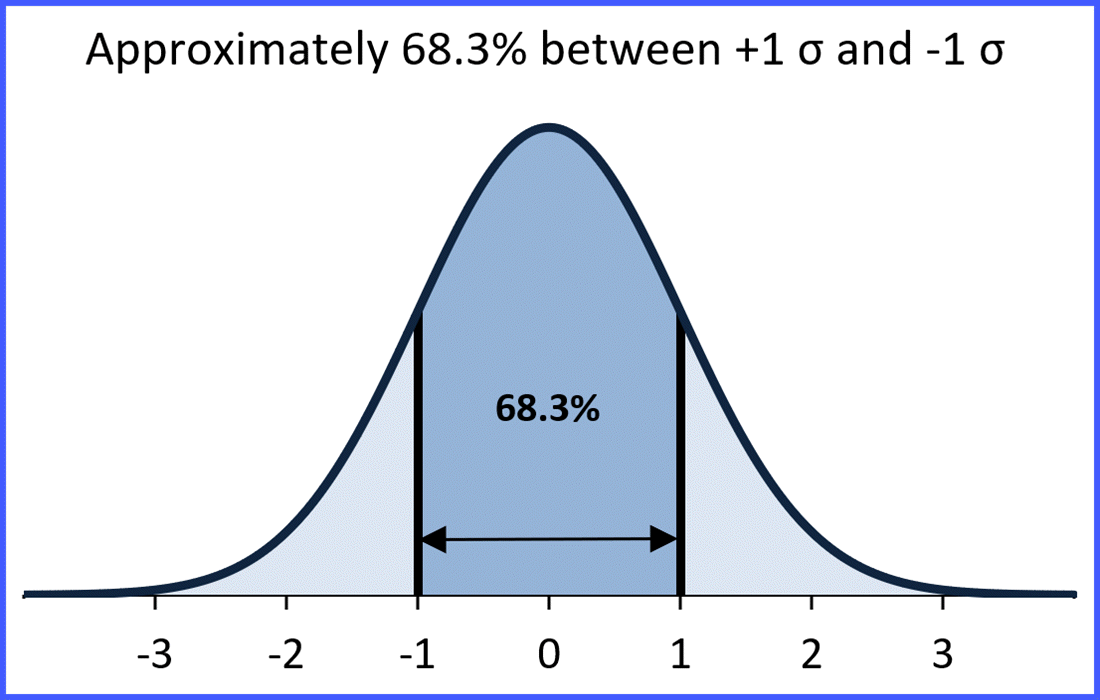And between +2 and -2 standard deviations, σ, we have approximately 95.4%.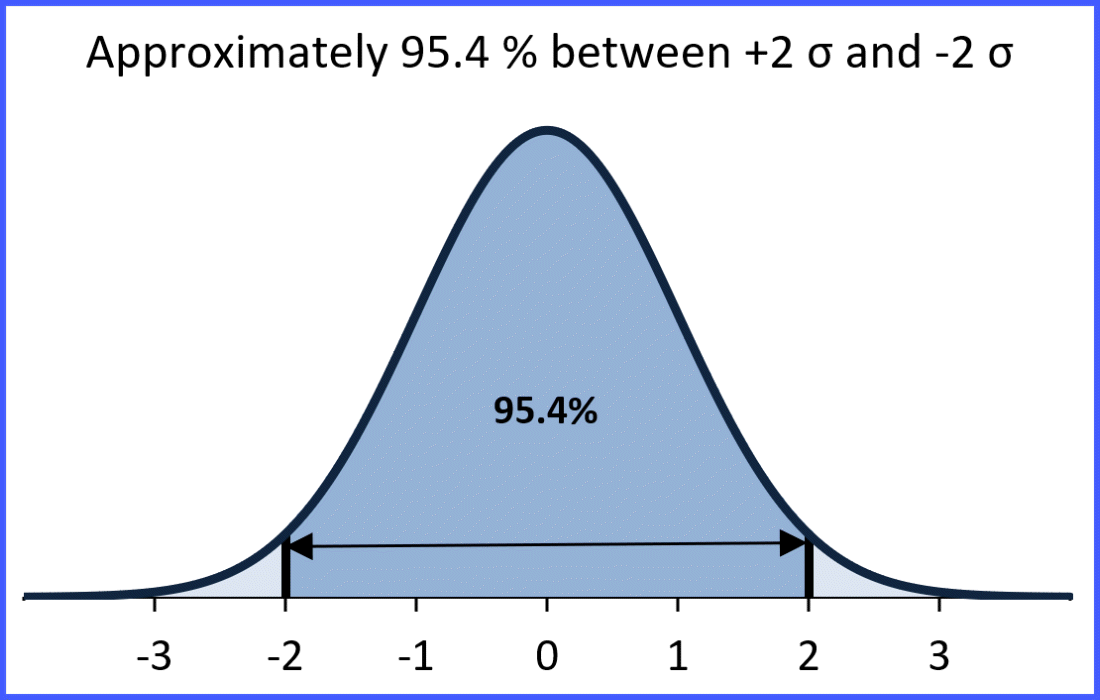Finally, between +3 and -3 σ, we have approximately 99.7%.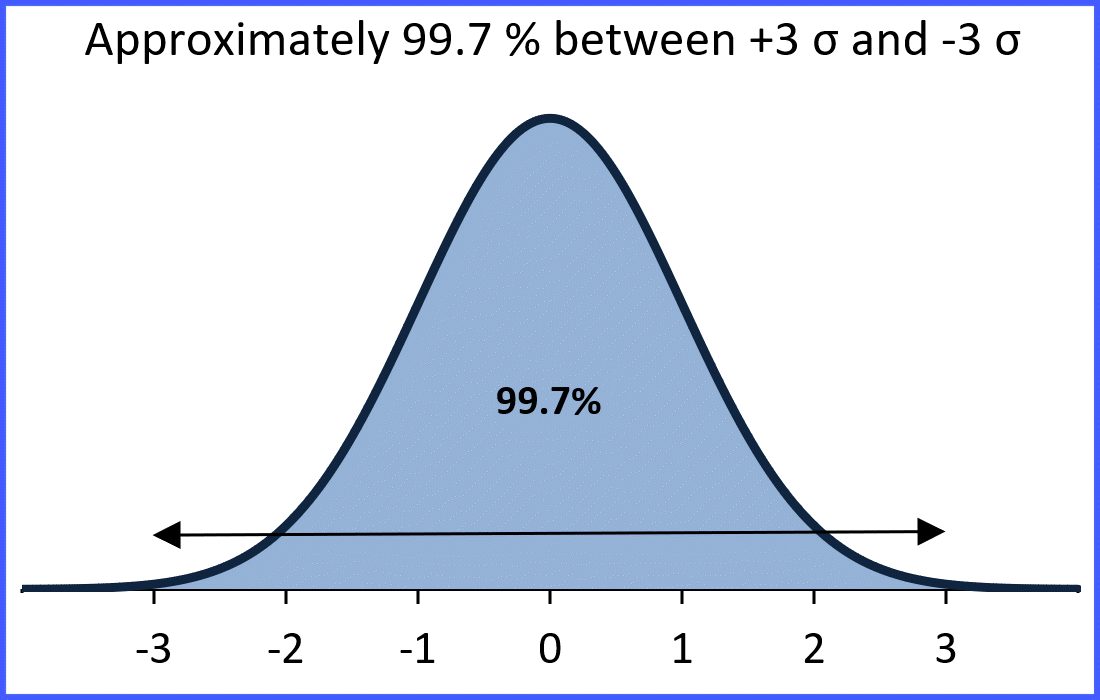Because there must be 100% of the data under the curve, that tells us there must be 100 – 99.7 = 0.3% split between the two tails beyond +3 and -3. Thus, there is approximately 0.15% to the right of +3 and 0.15% to the left of -3 σ.

#### How can we use the Empirical Rule?

We can use the Empirical Rule to find percentages and probabilities.

##### Example 1: IQ Scores have a bell-shaped distribution with a mean of 100 and a standard deviation of 15. What percentage of IQ scores are between 70 and 130?

Solution: 130 minus the mean of 100 = 30, which is 2 times 15, the standard deviation. Thus, 130 is 2 standard deviations to the right of the mean. 100 – 70 = 30 which is 2 times 15. Thus, 70 is 2 standard deviations to the left of the mean. Since 70 to 130 is within 2 standard deviations of the mean, we know that about 95.4% of the IQ scores would be between 70 and 130.In this example, we calculated the z-scores of the two IQ’s of interest. Here is the formula for finding a z-score which you should remember.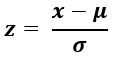The individual value we are interested in is “x.”  The mean of the population is the Greek letter Mu, μ. And the standard deviation of the population is Sigma, σ.

Here is the problem solved by first finding the z-scores of the two IQ’s we are interested in:Note: you can have + and – z-scores.

Converting an x value of interest into a z-score allows you to more easily use the Empirical Rule because the z-score is the number of standard deviations from the mean.

##### Example 2: What is the probability a randomly selected individual will have an IQ between 85 and 115?

Solution: Because the area under the bell curve represents 100% of the possible data points, it also represents probability. Recall that the probability of an event is the number of ways the event can happen divided by the total number of outcomes. Thus, an area under the bell curve equal to 10% is also a 10% probability of “x” falling in that area.

First, find the z-scores of the two IQs of interest:So, we need the area under the curve between  z = +1 and z = -1

Thus, there is about 68.3% probability a randomly selected individual will have an IQ between 85 and 115.

##### Example 3: What is the probability a randomly selected individual will have an IQ greater than 115?

Looking at the Empirical Rule graph above, we want the probability of an IQ score to the right of, greater than, 115, because that represents all the IQ scores above 115. That means we are interested in IQs with z scores  greater than +1. We know that about 68.3% of the scores are between -1 and +1 z. Because the bell curve is symmetrical, that gives us 100 – 68.3 = 31.7% split evenly between the two gold areas, Area 1 and Area 2. Thus, the probability of an individual having an IQ greater than 115 is Area 2, which is 31.7 divided by 2  = 15.85 or about 16%.

And by extension, the probability of a randomly selected individual having an IQ score less than 85 is also about 16%, which is Area 1.

Here is another image of the Empirical Rule that may help you solve z-score probability problems.

##### Example 4: What is the probability of an individual having an IQ score greater than 130?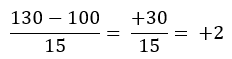Thus, an IQ greater than 130 would have a z-score greater than +2. To find the probability, just add the areas to the right of +2:

2.1 + 0.15 = 2.25% or about 2.3%

##### Example 5: What is the probability of an individual having an IQ score greater than 115?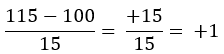Add the areas to the right of +1 z: 13.6 + 2.1 + 0.15 = 15.85 or about 16%.

##### Example 6: What is the probability of an individual having an IQ score greater than 85?Add the areas to the right of – 1 z: 34.1 + 34.1 + 13.6 + 2.1 + 0.15 = 84.05 or about 84%.

##### Example 7: What is the probability of an individual having an IQ score less than 130?Add the areas to the left of + 2 z: 13.6 + 34.1 + 34.1 + 13.6 + 2.1 + 0.15 = 97.65 or about 98%.

Or because the bell curve is symmetrical, add the areas to the right of +2 and subtract from 100%.

2.1 + 0.15 = 2.25.    100 – 2.25 = 97.75 or about 98%.

#### Remember:

The Empirical Rule gives approximate values, and the values in the graph are rounded off. So, you would look for the closest answer on a multiple-choice quiz.

### 1 thought on “Empirical Rule and z-score Probability”

This site uses Akismet to reduce spam. Learn how your comment data is processed.